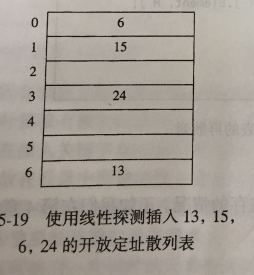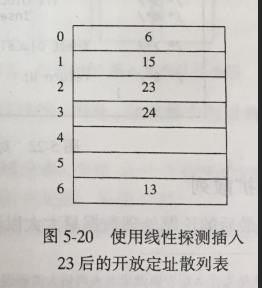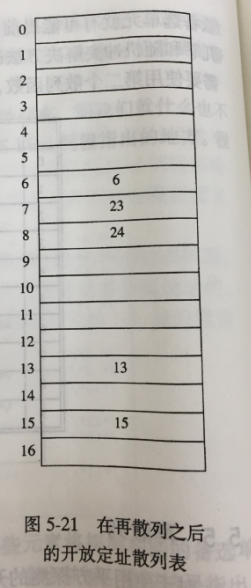# 【数据结构与算法分析】第五章 散列

## 1.散列函数

typedef unsigned int Index;
Index Hsh(const char *key,int TableSize)
{
unsigned  int HashVal=0;
while(*key!=0)
HashVal+=*key++;
return HashVal % TableSize;
}

Index Hash(const char *key,int TableSize)
{
unsigned  int HashVal=0;
while(*key!=0)
HashVal=(HashVal<<5)+*key++;//向左移动5位，即乘以32
return HashVal % TableSize;
}

## 2.分离链接法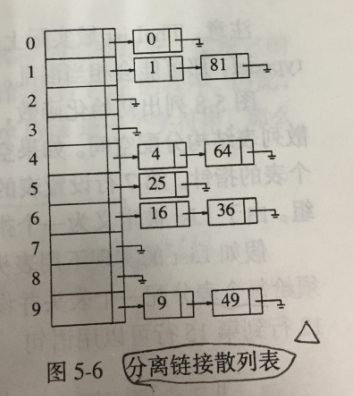#ifndef _Hash_H
struct ListNode;
typedef struct ListNode *Position;
struct HashTb;
typedef struct HashTb *HashTable;
HashTable InitializeTable(int TableSize);
void DestroyTable(HashTable H);
Position Find(ElementType Key,HashTable H);
void Insert(ElementType Key,HashTable H);
ElementType Retrieve(Position P);
#endef
struct ListNode
{
ElementType Element;
Position Next;
}
typedef Position List;
struct HashTb
{
int TableSize;
List *TheLists;
}

HashTable InitializeTable(int TableSize)
{
HashTable H;
int i;
if(TableSize < MinTableSize)
{
Error("Table size too small");
return NULL;
}
H=malloc(sizeof(struct HashTb));//分配列散表空间
if(H=NULL)
FatalError("out of space");
H->TableSize=NextPrime(TableSize);//设置表的大小为一个素数
H->TheLists=malloc(sizeof(List)*H->TableSize);//分配链表的空间
if(H->TheLists==NULL)
FatalError("out of space");
for(i=0;i<H->TableSize;i++)//分配每个链表的表头空间
{
H->TheLists[i] = malloc(sizeof(struct ListNode));
if(H->TheLists[i]==NULL)
FatalError("out of space");
else
H->TheLists[i]->Next = NULL;
}
return H;
}

Position Find(ElementType Key,HashTable H)
{
Posion P;
List L;
L=H->TheLists[Hash(key,H->TableSize)];//散列值相同的表，L为表头
P=L->Next;//表的第二个元素的位置
while(P!=NULL&&P->Element!=key)//如果有第二个元素
P=P->Next;
return P;//返回P的值，为NULL则无重复，否则重复
}

void Insert(ElementType Key,HashTable H)
{
Position Pos,NewCell;
List L;
Pos = Find(Key,H);//查看是否存在散列值
if(Pos==NULL)//散列值不存在
{
NewCell=malloc(sizeof(struct ListNode));//分配链表中一个元素的内存
if(NewCell==NULL)
FatalError("out of space");
else
{
L=H->TheLists[Hash(Key,H->TableSize)];//散列表下的一个链表，L为表头
NewCell->Next=L->Next;//
L->Next=NewCell;//向链表中插入一个值
NewCell->Element=Key;//向前插入，插入链表中的最前位置
}
}
}

## 3.开放定址法

#ifndef _HashQuad_H
typedef unsigned int Index;
typedef Index Position;
struct HashTb;
typedef struct HashTb *HashTable;
HashTable InitializeTable(int TableSize);
void DestroyTable(HashTable H);
Position Find(ElementType Key,HashTable H);
void Insert(ElementType Key,HashTable H);
ElementType Retrieve(Position P,HashTable H);
HashTable Rehash(HashTable H);
#endef
​
enum KindOfEntry{Legitimate,Empty,file};
struct HashEntry
{
ElementType Element;
enum KindOfEntry Info;
};
typedef struct HashEntry cell;
struct HashTb
{
int TableSize;
Cell *TheCells;
}

HashTable InitializeTable(int TableSize)
{
HashTable H;
int i;
if(TableSize < MinTableSize)
{
Error("tablesize too small");
return NULL;
}
H=malloc(sizeof(struct HashTb));//为散列表分配内存
if(H==NULL)
FatalError("out of space");
H->TableSize=NextPrime(TableSize);//设置散列表的大小为一个素数
H->TheCells=malloc(sizeof(Cell)*H->TableSize);//分配散列表的表项单元的数组
if(H->TheCell==NULL)
FatalError("out of space");
for(i=0;i<H->TableSize;i++)//将每个表项单元的数组设置为Empty
H->TheCells[i].Info=Empty;
return H;
}

### 3.1线性探测法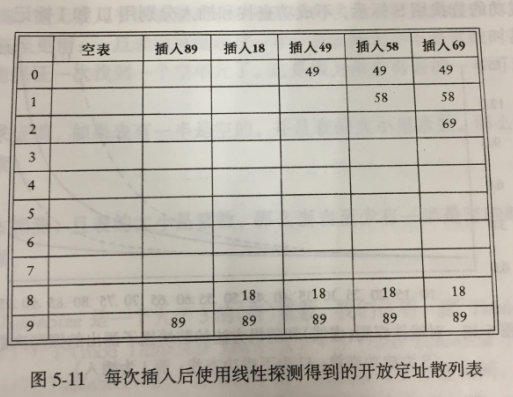### 3.2平方探测法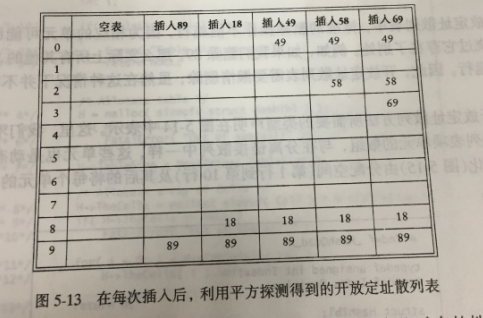Position Find(Element key,HashTable H)
{
Position CollitionPos;
int CollitionNum;
CollitionNum=0;
CollitionPos=Hash(key,H->TableSize);//返回散列值
while(H->TheCells[CollitionPos].Info!=Empty&&H->TheCells[CollitionPos].Element!=key)//如果冲突,1次：CollitionPos=1，2次CollitionPos=4，3次：CollitionPos=9...
{
CollitionPos +=2 * ++CollitionNum - 1;//公式：F(i)=F(i-1)+2i-1
if(CollitionPos>=H->TableSize)
CollitionPos-=H->TableSize;
}
return CollitionPos;//返回key应插入的位置
}

void Insert(ElementType key,HashTable H)
{
Position Pos;
Pos=Find(key,H);//Pos为应该插入的位置
if(H->TheCells[Pos].Info!=Legitimate)//合法
{
H->TheCells[Pos].Info=Legistimate;
H->TheCells[Pos].Element=key;
}
}

### 3.3双散列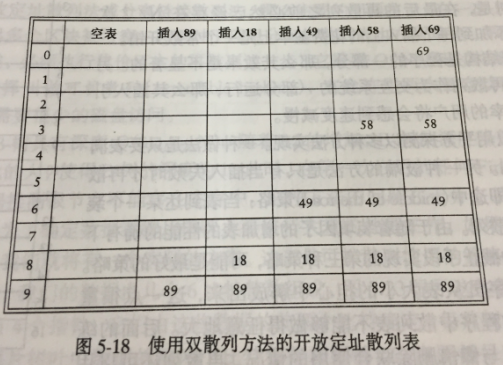## 4.再散列